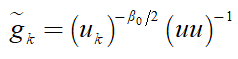# {convex} .* {convex}

I want to write this formula in code.In CVX, I wrote the following code.

g_k=pow_p(u_k,-FL/2)*pow_p(uu,-1);

u_k and uu are variables. They are all positive numbers.
Matlab said Disciplined convex programming error:
Cannot perform the operation: {convex} .* {convex}

z = feval( oper, x, y );

g_k=pow_p(u_k,-FL/2)*pow_p(uu,-1);

I tried to write like
g_k=inv_pos(pow_abs(u_k,FL/2)*uu);
But there are still mistakes.

Is g_k convex? you need to make sure it is convex. if it is not, then you can never write it in CVX. if it is, please show us why.

That looks like basically a multiplication (or division) of variables (expressions), which is non-convex. Your only hope of using this in CVX is if there is some reformulation to not form g_k explicitly, but rather refromulate or “smudge” it into something else; but that seems rather unlikely.

Your posts repeatedly violate the guidance therein. You will find elaboration on the guidance from @jackfsuia in that link.

I think gk is convex. I calculated that the Hessian matrix of the function is positive semidefinite.

Replace {g_{k}} with {e^{-A}}. Then add the following constrains:

A\le \ln uu+\left( {{\beta _0}/2} \right)\ln {u_k}

It might work.